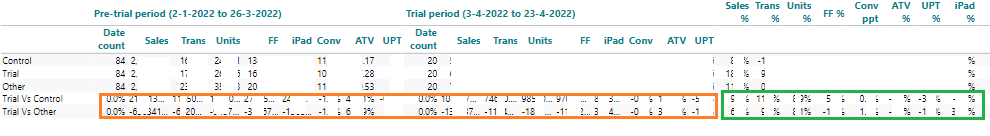# Column Order Number to be used in Row Calculation

I want to create a row which numbers all column e.g. 1,2,3,4 etc. across all dimension and calculations so I can then reference it in another row i.e. below I do not want to apply calculation to the orange box but do for the Green box so I end up with something like:

If Col <10 then 0 Else if Col = 11 then 1+1 if Col > 11 then 2+2 else 3+3 etc.

I can't seem to create a column number order for all columns, I thought I would create a row of 1 then another row summing from previous columns but bit stuck, any ideas?